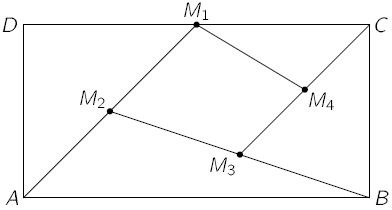## ksf-2015-st-27

In the rectangle $$ABCD$$ shown in the figure, $$M_1$$ is the midpoint of $$DC$$, $$M_2$$ is the midpoint of $$AM_1$$, $$M_3$$ is the midpoint of $$BM_2$$ and $$M_4$$ is the midpoint of $$CM_3$$.Question:

Find the ratio between the areas of the quadrilateral $$M_1M_2M_3M_4$$ and of the rectangle $$ABCD$$.